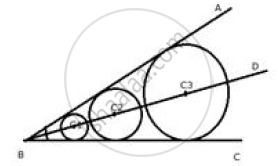# Describe:  The locus of the centres of all circles that are tangent to both the arms of a given angle. - Mathematics

Sum

Describe:

The locus of the centres of all circles that are tangent to both the arms of a given angle.

#### Solution

The locus of the centre of all circles whose tangents are the arms of a given angle is the bisector of that angle.Concept: Introduction of Loci
Is there an error in this question or solution?

#### APPEARS IN

Selina Concise Maths Class 10 ICSE
Chapter 16 Loci (Locus and Its Constructions)
Exercise 16 (B) | Q 15.6 | Page 241